# Use the SUM function to add up a column or row of cells in Excel

There are a variety of ways to add up the numbers found in two or more cells in Excel. This lesson shows you several methods including the SUM function and the Autosum button.

## Option One - simple addition

Let's say you have several cells that contain numbers, and you would like to add those numbers together:You can add up the values for Monday to Friday (from cell B2 through to B6) by typing the following formula into another cell in your spreadsheet. In this example, I've started typing the formula into cell B6. Notice how the cells in my formula have been highlighted as I typed my formula: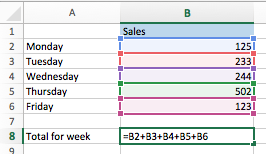When I press Enter, the formula will be stored in the cell, and the result of the formula will be displayed as shown in the example below:Note that if one or more of the cells are empty, or contain text rather than a number, Excel will simply ignore those values when calculating the result.

Also note that Excel doesn't mind if you type the formula in lower case as shown below - Excel will automatically convert each letter to uppercase when you press Enter:

In other words, typing this: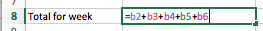is the same as typing this:The great thing about adding up cells in this way is that the formula will always show the current value of the sum of these cells. Any time you change one of the values in cells B2, B3, B4, B5 or B6, the value in B8 will change immediately to show the correct sum of these cells.

A faster way to add up the values in several cell is to follow these steps:

1. Click on the cell where you want the result of the calculation to appear.
2. Type = (press the equals key to start writing your formula)
3. Click on the first cell to be added (B2 in this example)
4. Type + (that's the plus sign)
5. Click on the second cell to be added (A3 in this example)
6. Type + again, and the next cell to be added.]
7. Repeat until all cells to be added have been clicked.
8. Press Enter.

This will create the same formula as shown in the example above without you needing to manually type the whole formula.

Of course, this approach to adding up cells becomes difficult to manage once you have a large number of cells to be added. For that reason, you may prefer the next method of adding up cells in Excel, which uses a function to do the addition for you.

## Option Two - use the SUM() function to add up a range of cells

The SUM() function is a much more efficient way to add up cells. It can be used to add up individual cells, as we did in the last example. However, it also allows you to add up a range of cells simply by specifying the first and last cell in a range of cells to be added up. The SUM() function will then add up the values in all the cells from the start to the end of the range. It is particularly powerful because it can be used to write a simple formula add up hundreds or thousands of cells .

If we look at our earlier example, you could use SUM() as shown in the following formula, which would achieve the same result as our earlier example:Notice how the cells included in the formula are highlighted - this is a useful way to check whether your formula is using the correct range of cells for its calculations.

This formula adds up all the cells from B2 to B6 inclusive. This method could be used just as easily to add up several thousand cells, as shown in this example: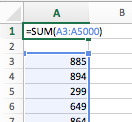You could also use it to add up a row of numbers, such as this example: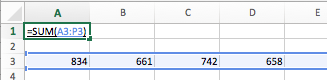You can also use it to add up cells in more than one row and more than one column at a time, as shown in this example: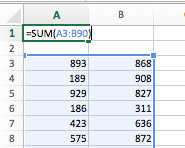Finally, you can add up more than one range of cells at at time. Our earlier SUM() examples have only added up one range at a time. This example adds up several ranges at once. All the cells in all the ranges are added together to give a grand total: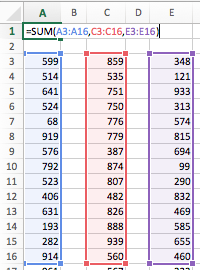As you can see, this formula specifies three separate ranges, each of which will be included in the same SUM() calculation.

This brings us to one final example of how to use the SUM() function. Whilst there are no wrong ways to add up cells in Excel, here is an example of a a SUM formula that actually takes longer to create than it should. This is a common mistake made by new Excel users, and it can quickly become a bad habit:## Our Comment Policy.

Add a comment to this lesson

### Calc doesn't allow to hide/show rows/columns

Select 2 adjacent rows of hide row then right click on it and click show .Then it displays hide row

### I think,its related with only

I think,its related with only Libre office

### Where is Autosum ??

This isn't helpful if we can't find it. I have version 11.6.6 and can't find anything called "autosum". -.-

### Auto sum

When you can't find something like auto sum, open up "Excel help" and type it in. I have learned a great deal via their help links. Or just try mousing over things on the ribbon to see what they do.

### Autosum

Thanks, that's exactly what I needed in five minutes!

I found this site very helpful, thank you

### Directions on Cell function

I was checking on how to enter SUM functions for selected cells. The directions were very clear and helpful EXCEPT they never gave the actual function you are to use. Ex: The following is from your help center:

Option Two - use the SUM() function to add up a range of cells
The SUM() function is a much more efficient way to add up cells. It can be used to add up individual cells, as we did in the last example. However, it also allows you to add up a range of cells simply by specifying the first and last cell in a range of cells to be added up. The SUM() function will then add up the values in all the cells from the start to the end of the range. It is particularly powerful because it can be used to write a simple formula add up hundreds or thousands of cells .

If we look at our earlier example, you could use SUM() as shown in the following formula, which would achieve the same result as our earlier example:

My response:
The example where you actually can see the formula was very small and difficult to read and in lighter font. Wouldn't it be easier to use an example of the formula you are recommending, ex: Write in larger font: " To Sum a series of cells, enter this formula in the cell you want: =SUM (C2:C7) " ?
I was able to figure it out, but it was hard to see in the visual example you gave.
However, I AM grateful for the help, and now know how to do it.

### Using variables as parameters on R1C1 Sum formulas.

I'm trying to move the Total down as the number of entries is increased. So if I start in cell(1,1) the total after the first entry will be in cell (2,1). After my second entry the total will move to cell(3,1) and so on.